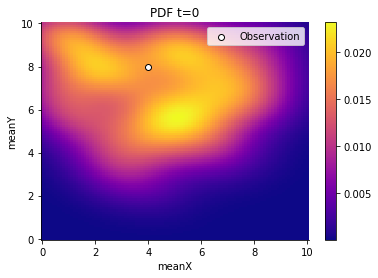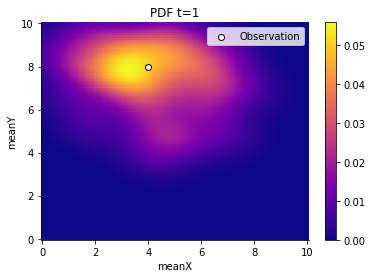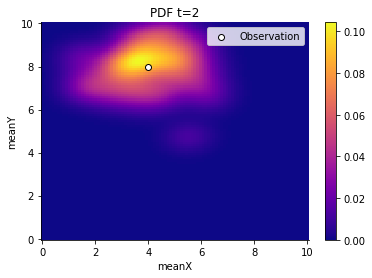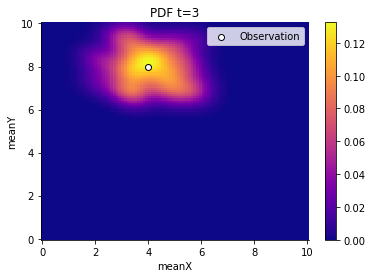# Using R via rpy2

This example illustrates how to use models, summary statistics and distance functions defined in R. We’re assuming you’re already familiar with the basic workings of pyABC. If not, consult the other tutorial examples.

Download this notebook Using R with pyABC.

In this example, we’re introducing the new class pyabc.external.r.R which is our interface with R. We use this class to to load an external R script.

:

# install if not done yet
!pip install pyabc[r] --quiet

:

%matplotlib inline
from pyabc.external.r import R

r = R("myRModel.R")

/home/yannik/pyabc/pyabc/external/r/r_rpy2.py:81: UserWarning: The support of R via rpy2 is considered experimental.
warnings.warn("The support of R via rpy2 is considered experimental.")


Note

R("myRModel.R") does, amongst other things, the equivalent to R’s source("myRModel.R"). That is, the entire script is executed with all the possible side effects this might have.

You can download the file here: myRModel.R. But now, let’s have a look at it.

:

r.display_source_ipython()

:

# Blue print for pyABC parameter inference runs.
# A proposal of how to structure an R file for usage with pyABC

#' The model to be simulated.
#' In this example, it is just a multivariate normal
#' distribution. The number of parameters depends on the
#' model and can be arbitrary. However, the parameters
#' should be real numbers.
#' The return type is arbitrary as well.
myModel <- function(pars){
x <- rnorm(1) + pars$meanX y <- rnorm(1) + pars$meanY
c(x,y)  # It is not important that it is a vector.
}

#' Calculates summary statistics from whatever the model returns
#'
#' It is important that the summary statistics have names
#' to store them correctly in pyABC's database.
#' In many cases, the summary statistics function might just
#' pass through the result of the model function if the
#' summary statistics calculation is already
#' done there. Splitting summary statistics and the model
#' makes most sense in a model selection scenario.
#'
#' @param modelResult The data simulated by the model
#' @return Named list of summary statistics.
mySummaryStatistics <- function(modelResult){
list(x=modelResult,
y=modelResult,
mtcars=mtcars,  # Can also pass data frames
cars=cars,
arbitraryKey="Some random text")
}

#' Calculate distance between summary statistics
#'
#' @param sumStatSample The summary statistics of the sample
#' proposed py pyABC
#' @param sumStatData The summary statistics of the observed
#'        data for which we want to calculate the posterior.
#' @return A single float
myDistance <- function(sumStatSample, sumStatData){
sqrt((sumStatSample$x - sumStatData$x)^2
+ abs(sumStatSample$y - sumStatData$y)^2)
}

# We store the observed data as named list
# in a variable.
# This is passed by pyABC as to myDistance
# as the sumStatData argument
mySumStatData <- list(x=4, y=8, mtcars=mtcars, cars=cars)

# The functions for the model, the summary
# statistics and distance
# have to be constructed in
# such a way that the following always makes sense:
#
# myDistance(
#   mySummaryStatistics(myModel(list(meanX=1, meanY=2))),
#   mySummaryStatistics(myModel(list(meanX=2, meanY=2))))


We see that four relevant objects are defined in the file.

• myModel

• mySummaryStatistics (optional)

• myDistance

• mySumStatData

The names of these do not matter. The mySummaryStatistics is actually optional and can be omitted in case the model calculates the summary statistics directly. We load the defined functions using the r object:

:

model = r.model("myModel")
distance = r.distance("myDistance")
sum_stat = r.summary_statistics("mySummaryStatistics")


From there on, we can use them (almost) as if they were ordinary Python functions.

:

import pyabc
from pyabc import ABCSMC, RV, Distribution

pyabc.settings.set_figure_params('pyabc')  # for beautified plots

prior = Distribution(meanX=RV("uniform", 0, 10), meanY=RV("uniform", 0, 10))
abc = ABCSMC(model, prior, distance, summary_statistics=sum_stat)

ABC.Sampler INFO: Parallelize sampling on 4 processes.


We also load the observation with r.observation and pass it to a new ABC run.

:

import os
from tempfile import gettempdir

db = "sqlite:///" + os.path.join(gettempdir(), "test.db")
abc.new(db, r.observation("mySumStatData"))

ABC.History INFO: Start <ABCSMC id=1, start_time=2021-11-18 15:44:06>

:

<pyabc.storage.history.History at 0x7fef803bfdc0>


We start a run which terminates as soon as an acceptance threshold of 0.9 or less is reached or the maximum number of 4 populations is sampled.

:

history = abc.run(minimum_epsilon=0.9, max_nr_populations=4)

ABC INFO: Calibration sample t = -1.
ABC INFO: t: 0, eps: 4.33680673e+00.
ABC INFO: Accepted: 100 / 240 = 4.1667e-01, ESS: 1.0000e+02.
ABC INFO: t: 1, eps: 2.94456492e+00.
ABC INFO: Accepted: 100 / 256 = 3.9062e-01, ESS: 9.7804e+01.
ABC INFO: t: 2, eps: 1.88636339e+00.
ABC INFO: Accepted: 100 / 289 = 3.4602e-01, ESS: 8.9331e+01.
ABC INFO: t: 3, eps: 1.23695847e+00.
ABC INFO: Accepted: 100 / 471 = 2.1231e-01, ESS: 8.4991e+01.
ABC INFO: Stop: Maximum number of generations.
ABC.History INFO: Done <ABCSMC id=1, duration=0:00:09.465072, end_time=2021-11-18 15:44:15>


Lastly, we plot the results and observe how the generations contract slowly around the oserved value. (Note, that the contraction around the observed value is a particular property of the chosen example and not always the case.)

:

from pyabc.visualization import plot_kde_2d

for t in range(history.n_populations):
df, w = abc.history.get_distribution(0, t)
ax = plot_kde_2d(
df,
w,
"meanX",
"meanY",
xmin=0,
xmax=10,
ymin=0,
ymax=10,
numx=100,
numy=100,
)
ax.scatter(
, , edgecolor="black", facecolor="white", label="Observation"
)
ax.legend()
ax.set_title(f"PDF t={t}")And we can also retrieve summary statistics such as a stored DataFrame, although the DataFrame was acutally defined in R.

:

history.get_weighted_sum_stats_for_model(m=0, t=1)["cars"].head()

:

speed dist
1 4.0 2.0
2 4.0 10.0
3 7.0 4.0
4 7.0 22.0
5 8.0 16.0

## Dumping the results to a file format R can read

Although you could query pyABC’s database directly from R since the database is just a SQL database (e.g. SQLite), pyABC ships with a utility for facilitate export of the database. Use the abc-dump utility provided by pyABC to dump results to file formats such as csv, feather, html, json and others. These can be easiliy read in by R. See Exporting pyABC’s database for how to use this utility.

Assume you dumped to the feather format:

abc-export --db results.db --out exported.feather --format feather


You could read the results in with the following R snippet

install.packages("feather")
install.packages("jsonlite")

library("feather")
library("jsonlite")